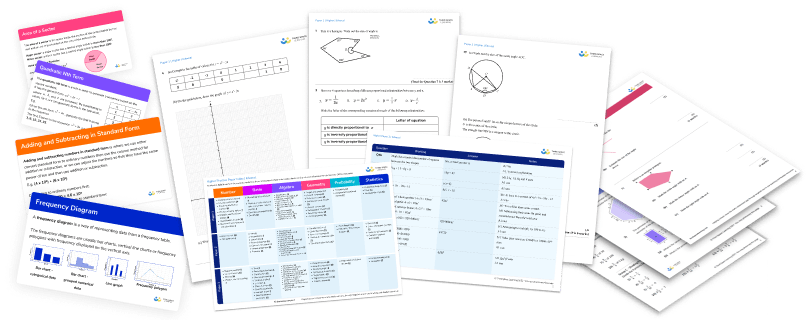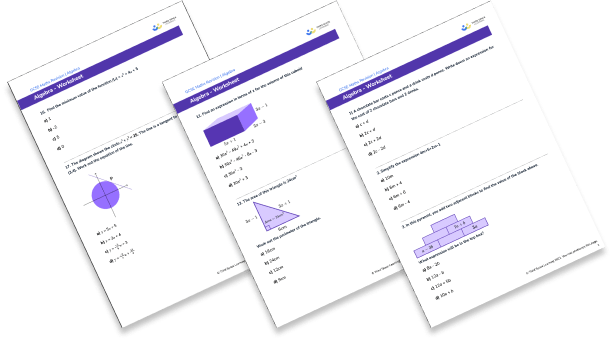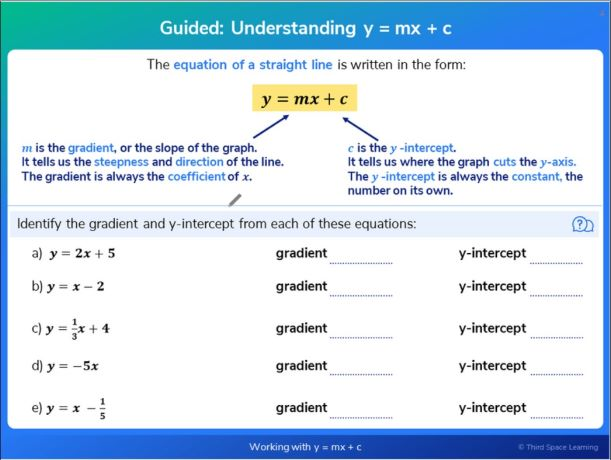Teaching support from the UK’s largest provider of in-school maths tuition.one to one lessonsschools supported

Shape confident mathematicians

One to one tuition designed to build confidence and engagement in an interactive low-stakes environment

FREE secondary maths resources

Here you can find all of our free secondary resources; explore the collections or navigate to each section to filter resources by topicExplore all resources
Secondary Worksheets & Practice Questions

Shape confident mathematicians

One to one tuition designed to build confidence and engagement in an interactive low-stakes environment

# 15 Algebra Questions And Practice Problems (KS3 & KS4): Harder GCSE Exam Style Questions Included

Algebra questions involve using letters or symbols to represent unknown values or values that can change. Here you will find 15 algebra questions to test your knowledge and show you the different ways that algebra can be used to solve a problem to find an unknown value or to make generalisations.

Free GCSE maths revision resources for schools
As part of the Third Space Learning offer to schools, the personalised online GCSE maths tuition can be supplemented by hundreds of free GCSE maths revision resources including:
GCSE maths past papers
GCSE maths worksheets
GCSE maths questions
GCSE maths topic list

### Algebra in KS3 and KS4

There are many topics and techniques within algebra. In KS3 we learn to write and manipulate basic algebraic expressions and linear equations. In KS4 we develop these techniques to allow us to deal with more complicated algebra problems such as ones that involve quadratic equations or a system of equations.

### How to solve algebraic questions

When you are presented with an algebraic problem it is important to establish what you are being asked to do. Here are some of the key terms along with what they mean:

• Solve the equation – find out the value of the unknown
• Substitute – put the values you have been given into the algebraic expression
• Simplify – collect together like terms to make the expression or equation look simpler
• Expand brackets – multiply out the brackets
• Factorise – put into brackets
• Make x the subject – rewrite the equation in the form x =…..

Remember, when working with algebra, we must still apply BODMAS / BIDMAS. i.e. Brackets, Indices (powers, exponents, square roots), Division, Multiplication, Addition, Subtraction.

When working with algebraic expressions and equations we must consider carefully which operations to deal with first.Download this 15 Algebra Questions And Practice Problems (KS3 & KS4) WorksheetDownload Free Now!

### Algebra in KS2

The ideas of writing and simplifying expressions, solving equations and substitution are introduced in KS2. Here are some example KS2 algebra questions:

#### Algebra questions KS2

1. A chocolate bar costs c pence and a drink costs d pence. Write down an expression for the cost of 2 chocolate bars and 2 drinks.

c+d2c+d2c+2d2c-2d2 chocolate bars would cost 2 lots of c, or 2c, and 2 drinks would cost 2 lots of d, or 2d.

2. Simplify the expression 4m+5+2m-1

10m6m+46m+66m-4We need to collect together like terms here so 4m + 2m = 6m and 5 – 1 = 4 (watch out for the negative).

### Algebra questions KS3

In KS3 we learn a variety of different algebra techniques to answer algebra questions and to practise problem solving with algebra. These include:

• Simplifying algebraic expressions
• Expanding brackets and factoring
• Forming algebraic equations from word problems
• Solving algebraic equations and inequalities
• Substituting into expressions
• Changing the subject of an equation
• Working with real life graphs and straight line graphs
• SequencesA slide guiding pupils through the equation of a straight line from Third Space Learning’s online intervention.

#### Algebra questions KS3: basic algebra

1. In this pyramid, you add two adjacent blocks to find the value of the block above.What expression will be in the top box?

8a-2b12a-b12a+5b10a+b2.  Brian is a window cleaner. He uses the following formula to calculate the amount to charge his customers:

Charge = £20 + 4n

Where n is the number of windows a house has.

If a house has 7 windows, how much would Brian charge?

£24£67£48£27In this question, n is 7 so we can substitute 7 into the formula.

Charge = £20 + 4 × 7

Charge = £48

3. The area of a rectangle is 4x-6.Which of the following pairs could be the length and width of the rectangle?

4x and 6x4 and x-62 and 2x-32x and 2x-3There are two ways of attempting this question. We know that area \;of \;a \;rectangle = length × width so we could multiply each pair together to see which pair makes 4x − 6.

Alternatively, if we factorise 4x − 6 we get 2(2x − 3) meaning the sides could be 2 and 2x − 3.

4.  The formula for changing degrees Celsius to degrees Fahrenheit is

F=\frac{9C}{5}+32

Rearrange this formula to make C the subject.

C=\frac{5(F-32)}{9}C=\frac{5F-32}{9}C=\frac{5F}{9}-32C=5F-\frac{32}{9}\begin{aligned} F&=\frac{9C}{5}+32 \hspace{3cm} &\text{subtract 32}\\\\ F-32&=\frac{9C}{5} &\text{multiply by 5}\\\\ 5(F-32)&=9C &\text{divide by 9}\\\\ \frac{5(F-32)}{9}&=C \end{aligned}

#### Algebra questions KS3: forming and solving equations

5. Work out the size of the smallest angle.20^{\circ}26^{\circ}8^{\circ}34^{\circ}The angles in a triangle add up to 180^{\circ} therefore we can write

4x+2x-10+3x-8=180

Now we have an equation we can solve.

\begin{aligned} 9x-18&=180 \hspace{3cm} &\text{add } 18\\ 9x&=198 &\text{divide by } 9\\ x&=22^{\circ} \end{aligned}

The angles are :

\begin{aligned} 4\times22&=88^{\circ}\\ 2\times22 -10&=34^{\circ}\\ 3\times22 -8&=58^{\circ} \end{aligned}

The smallest angle is 34^{\circ} .

6.  Jamie’s dad is 4 times older than Jamie. In 14 years time, Jamie’s dad will be twice the age of Jamie.

What is the sum of Jamie’s age now and Jamie’s dad’s age now?

70422235To solve this we need to write an equation.

Let Jamie’s age now be x . Then Jamie’s dad’s age is 4x .

In 14 years time Jamie’s age will be x + 14 and Jamie’s dad’s age will be 4x + 14 .

Since we know Jamie’s dad’s age will be two times Jamie’s age, we can write

4x+14=2(x+14)

Now we have an equation we can solve.

\begin{aligned} 4x+14&=2(x+14) \hspace{3cm} &\text{expand the brackets}\\ 4x+14&=2x+28 \hspace{3cm} &\text{subtract 2x}\\ 2x+14&=28 \hspace{3cm} &\text{subtract 14}\\ 2x&=14 \hspace{3cm} &\text{expand the brackets}\\ x&=7 \hspace{3cm} \end{aligned}

Jamie is currently 7 years old meaning his dad is 28 years old. The sum of their ages is 35 .

#### Algebra questions KS3: graphs

7.  Which of the following lines passes through the point (2, 5)?

y=2x-1y=2x+1y=4x-2y=2x+5At the point (2, 5), x is 2 and y is 5. We can check which equation works when we substitute in these values:

### Algebra questions KS4

Algebra is studied extensively in the GCSE and IGCSE curriculum.

In KS4 we build on the techniques learnt in KS3. Topics include:

• Expanding and factorising polynomials
• Solving simultaneous equations
• Inequalities
• Algebraic fractions
• Further work on graphs
• Functions

#### Algebra questions KS4: algebraic manipulation

8. Which of the following expressions has the smallest value when a=5 and b=-3?

\frac{1}{2}(a-b)abb^2b − 4a9. Find an expression in terms of x for the volume of this cuboid30x^3-49x^2+4x+330x^3-46x^2-8x-330x^3-330x^3+3Volume of a cuboid = length × width × height

Volume = (5x+1)(2x-3)(3x-1)

Volume = (10x^2+2x-15x-3)(3x-1)

Volume = (10x^2-13x-3)(3x-1)

Volume = 30x^3-39x^2-9x-10x^2+13x+3

Volume = 30x^3-49x^2+4x+3

#### Algebra questions KS4: forming and solving equations

10. The area of this triangle is 24cm^2 .Work out the perimeter of the triangle.

16cm24cm12cm9cmThe area of a triangle is area = \frac{1}{2} × b × h.

If we fill in what we know we get:

\begin{aligned} 24&=\frac{1}{2}\times 6 \times (3x-1) \hspace{3cm} &\text{simplify}\\\\ 24&=3(3x-1) &\text{multiply out the brackets}\\\\ 24&=9x-3 &\text{add 3}\\\\ 27&=9x &\text{divide by 9}\\\\ x&=3 \end{aligned}

Since x = 3 , the side lengths are 6m, 8cm and 10cm .

The perimeter is 6 + 8 + 10 = 24cm .

11.  Solve the equation x+2-\frac{15}{x}=0

x=-2 or x=15x=-3 or x=5x=-5 or x=3x=-15 or x=2We can make this a bit easier by getting rid of the fraction involving x. We do this by multiplying each term by x.

\begin{aligned} x+2-\frac{15}{x}&=0 \hspace{3cm} &\text{multiply by x}\\\\ x^{2}+2x-15&=0 &\text{factorise}\\\\ (x+5)(x-3)&=0 &\text{solve}\\\\ &x=-5 \text{ or } x=3 \end{aligned}

12. At a theme park the Jones family purchased 2 adult tickets and 3 child tickets for £48 .  The Evans family purchased 3 adult tickets and 1 child ticket for £44

Calculate the cost of one child ticket.

£10£8£12£6We can write simultaneous equations to solve this.

2a+3c=48 (Equation 1)
3a+c=44 (Equation 2)

Multiply equation 2 by 3 to make the coefficients of c equal: 9a+3c=132 (Equation 3)

Subtract equation 1 from equation 3:
7a= 84
a=12

Substitute a into equation 3:
3×12+c=44
36+c=44
c=8

The cost of an adult ticket is £12 and a child ticket is £8 .

#### Algebra questions KS4: graphs

13.  Which of these lines is parallel to the line 2y = x + 7

3y=x+7y=2x-2y=\frac{1}{2}x-92y=3x+8For two lines to be parallel, their gradient must be equal.

If we rearrange 2y=x+7 to make y the subject we get y=\frac{1}{2}x+\frac{7}{2}.

14. Find the minimum value of the function f(x) = x^2+4x+5 .

1-250To find the minimum value we need to complete the square.

\begin{aligned} f(x)&=x^2+4x+5\\ f(x)&=(x+2)^2-4+5\\ f(x)&=(x+2)^2+1 \end{aligned}

The minimum value is 1. This occurs when (x+2) is 0.

15. The diagram shows the circle x^2+y^2=25 . The line is a tangent to the circle at the point (3,4) . Work out the equation of the line.y=5x+5y=3x+4y=\frac{-3}{4}x+3y=\frac{-3}{4}x+\frac{21}{4}To work out the gradient of the line we need to work out the gradient of the normal.

We know that the normal goes through the points (0, 0) and (3, 4) so we can calculate the gradient: \frac{4-0}{3-0}=\frac{4}{3}.

The gradient of the tangent will be \frac{-3}{4}.

We can now use y=mx+c . We know the tangent goes through the point (3, 4) and that it’s gradient is \frac{-3}{4} .

Therefore

\begin{aligned} 4&=\frac{-3}{4} \times 3 +c\\\\ 4&=\frac{-9}{4}+c\\\\ 4+\frac{9}{4}&=c\\\\ \frac{25}{4}&=c \end{aligned}

y=\frac{-3}{4}x+\frac{25}{4} \text{ or } 4y=-3x+25

#### Looking for more algebra questions and resources?

Third Space Learning’s free GCSE maths resource library contains detailed lessons with step-by-step instructions on how to solve algebra problems, as well as worksheets with algebra practice questions and more GCSE exam questions.

Take a look at the Algebra lessons today – more are added every week.

#### Looking for more KS3 and KS4 maths questions?

Try these:

Do you have students who need extra support in maths?
Every week Third Space Learning’s maths specialist tutors support thousands of students across hundreds of schools with weekly online 1-to-1 lessons and maths interventions designed to plug gaps and boost progress.

Since 2013 we’ve helped over 150,000 primary and secondary students become more confident, able mathematicians. Find out more about our GCSE Maths tuition or request a personalised quote for your school to speak to us about your school’s needs and how we can help.

Secondary school tuition designed to plug gaps and prepare KS3 and KS4 students for the challenges ahead.##### Beki Christian
Maths Teacher and Author
Beki has 11 years experience teaching secondary Maths. She is currently working as a Maths tutor as well as writing resources and blog posts for Third Space Learning.

Related Articles

x#### Download this 15 Algebra Questions And Practice Problems (KS3 & KS4) Worksheet